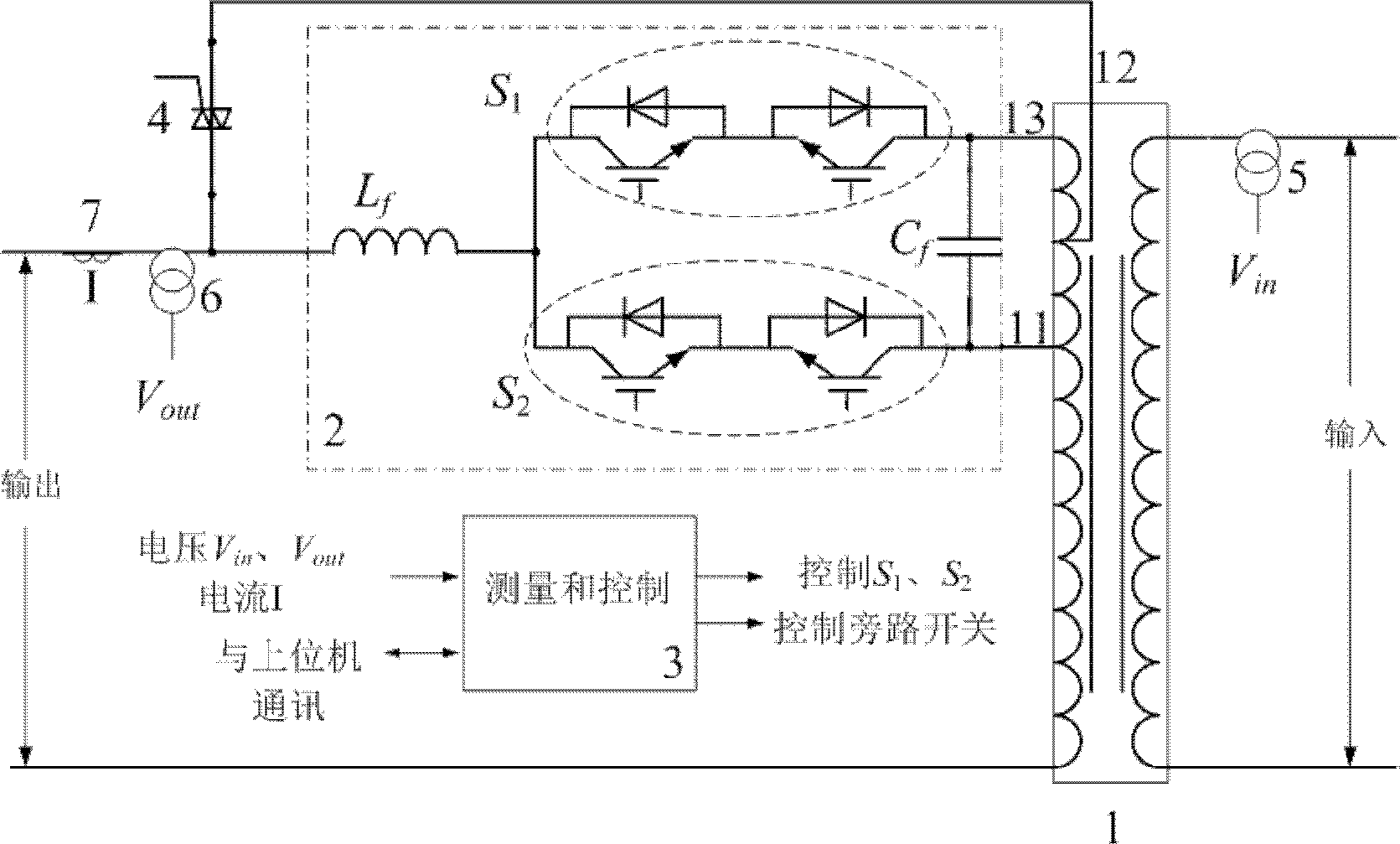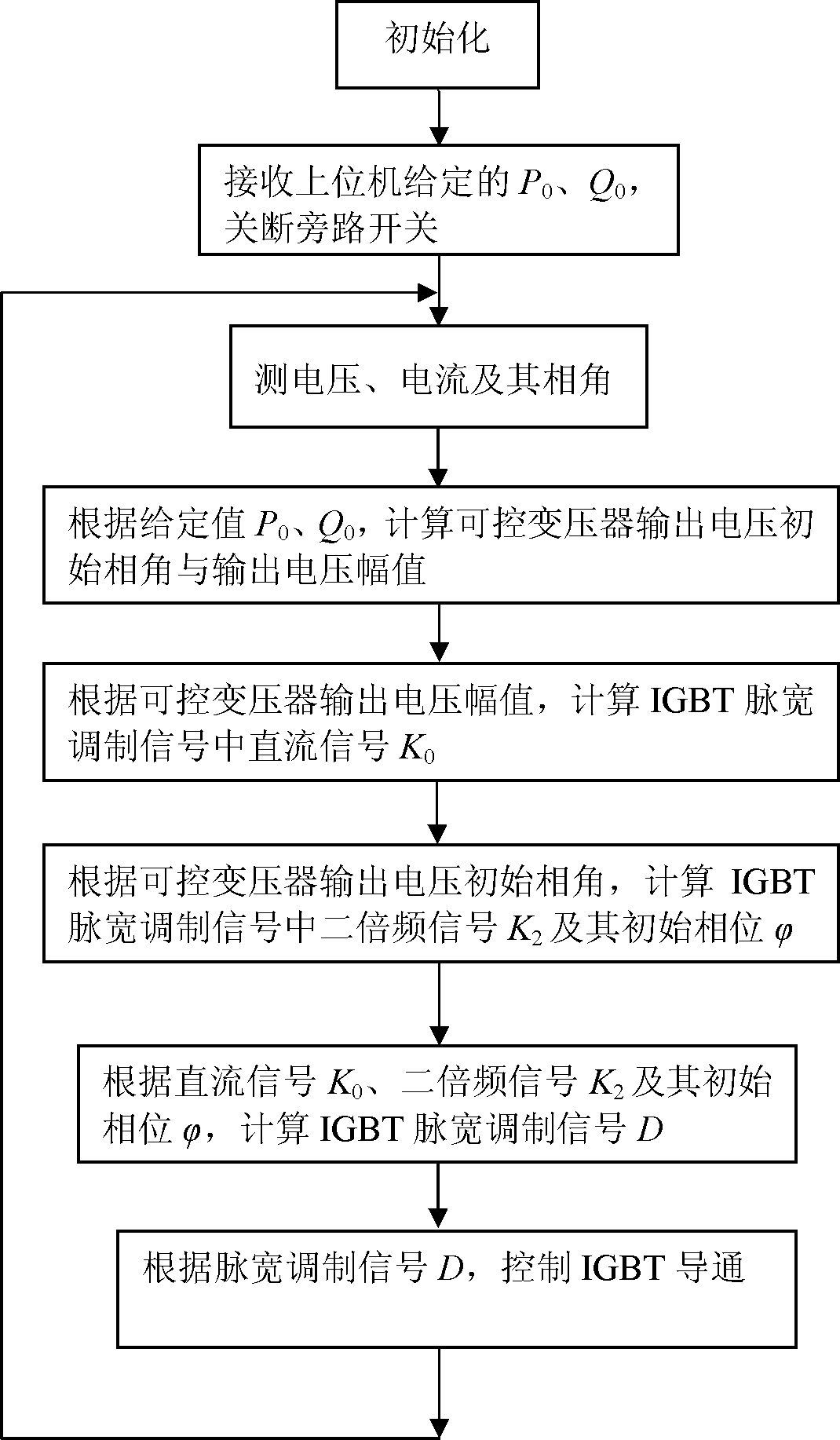# Output-side-switching-based dynamic power flow control device and control method for controllable transformer

## A dynamic power flow and control device technology, applied in the field of flexible power transmission, can solve problems such as large loss of FACTS devices, complex control structure, and system stability problems and limitations

Active Publication Date: 2012-02-15
SHANGHAI JIAO TONG UNIV
4 Cites 3 Cited by

## AI-Extracted Technical Summary

### Problems solved by technology

 However, the current FACTS technology also has great limitations: the cost of the FACTS device is high, and it is difficult to popularize and apply; there are adverse effects between the FACTS device and power equipment and other controllers; the loss of the FACTS device itself is large; The control structure and th...
View more

## Abstract

The invention discloses an output-side-switching-based dynamic power flow control device for a controllable transformer. The device consists of the controllable transformer, a power unit, a measurement and control module, a bypass switch, an input voltage transformer, an output voltage transformer and an output current transformer. In a dynamic power flow control method, the switching-on and switching-off of an output side/secondary tapping point of the controllable transformer are controlled by utilizing a fast switching-on/off power electronic switch, a frequency doubling signal is injectedinto a pulse width modulation signal of the power electronic switch of the controllable transformer by adopting a direct current signal, and the amplitude of the direct current signal and the amplitude and initial phase of the frequency doubling signal in the pulse width modulation signal are changed, thereby changing the phase and amplitude of output voltage of the controllable transformer and realize active power adjustment and passive power adjustment. The switching-on of the tapping point of the controllable transformer is controlled, thereby realizing adjustment control over the dynamic power flow of a power grid. The device and the method have the characteristics of low cost and high dynamic power flow control reliability.

Application Domain

Technology Topic

Power gridPulse width modulated +10

## Image

•••## Examples

• Experimental program(1)

### Example Embodiment

 The present invention will be further described below in conjunction with the embodiments and drawings, but the protection scope of the present invention should not be limited by this.
 See first figure 1 , figure 1 It is a schematic diagram of the structure of the dynamic power flow control device of the controllable transformer based on the output side switch of the present invention. As can be seen from the figure, a dynamic power flow control device for a controllable transformer based on an output side switch includes: controllable transformer 1, power unit 2, measurement and control module 3, bypass switch 4, input voltage transformer 5, output voltage Transformer 6 and output current transformer 7 constitute:
 The secondary side of the controllable transformer 1 includes a main connector 12 and a positive tap 13 and a negative tap 11;
 The power unit 2 consists of the first group of power tubes S 1 , The second group of power tubes S 2 , Filter inductor L f And filter capacitor C f Composition, the first group of power tubes S described in the power unit 2 1 And the second group of power tubes S 2 Both are composed of two insulated gate bipolar transistors connected in reverse series. The first group of power tubes S 1 And the second group of power tubes S 2 One end of the controllable transformer 1 is connected to the positive tap 13 and the negative tap 11 of the secondary side respectively, and the first group of power tubes S 1 And the second group of power tubes S 2 The other end is connected to the filter inductor L f One end of the filter inductor L f The other end is connected to the output power supply or load, and the filter capacitor C f Is connected between the positive tap 13 and the negative tap 11 on the secondary side of the controllable transformer 1, the first group of power tubes S 1 And the second group of power tubes S 2 The control terminal of is connected to the corresponding control terminal of the measurement and control module 3;
 The bypass switch 4 is connected between the main connector 12 on the secondary side of the controllable transformer 1 and the output power source or load;
 One side of the input voltage transformer 5 is connected to the primary input voltage main circuit of the controllable transformer, and the voltage signal output terminal is connected to the voltage signal input port of the measurement and control module 3;
 Said output voltage transformer 6, one side is connected to the main circuit of the secondary side output voltage of the controllable transformer, and the output end is connected to the voltage signal input port of the said measurement and control module 3;
 The output current transformer 7 is connected in series in the output main circuit of the controllable transformer, and its current signal output terminal is connected to the current signal input port of the measurement and control module 3;
 The control signal output terminal of the measurement and control module 3 is respectively connected to the first group of power tubes S of the power unit. 1 And the second group of power tubes S 2 The control terminal of is connected with the control terminal of the bypass switch 4, and the measurement and control module 3 is connected with the upper computer.
 The measurement and control module 3 is a digital signal processor, a single-chip microcomputer or a computer.
 Correct figure 2 In the system shown, the dynamic power flow control workflow of the controllable transformer based on the output side switch is as follows image 3 As shown, the above-mentioned dynamic power flow control device based on the controllable transformer of the output side switch is used to perform a power grid dynamic power flow control method, which includes the following specific steps:
 1) The measurement and control module 3 initializes the measurement and control, sends a signal to the bypass switch 4 to turn off the bypass switch, and receives the set value Q of the reactive power given by the upper computer 0 And the given value of active power P 0;
 2) The measurement and control module 3 receives the input voltage V inputted by the input voltage transformer 5, the output voltage transformer 6 and the output current transformer 7 respectively in , Output voltage V out , The output current I, the angle β between the output voltage and the output current, receive the remote grid voltage V Grid 2 Information and reactance value L of the transmission line:
 V Grid 2 =V 2 sin(ω 0 t+α), where V 2 Is its amplitude and α is its phase angle; calculate the measured active power P and reactive power Q according to the following formula:
 P = 1 2 V out I cos β
 Q = 1 2 V out I sin β ;
 3) According to active power P 0 And reactive power Q 0 ,According to the following formula, calculate the output voltage phase angle θ of the controllable transformer and the output voltage amplitude V out :
 P 0 = V 2 V out ω 0 L sin ( α - θ )
 Q 0 = V 2 2 - V 2 V out cos ( α - θ ) ω 0 L ,
 Among them: L is the reactance value of the transmission line;
 ω 0 The angular frequency corresponding to 50 or 60 Hz;
 V 2 Is the remote grid voltage V Grid 2 The amplitude of
 4) Calculate the pulse width modulation duty cycle D:
 ① According to the input voltage V of the controllable transformer in , Output voltage phase angle θ and amplitude V out ,Calculate the DC signal coefficient K in the pulse width modulation signal of the insulated gate bipolar transistor according to the following formula 0 , Double frequency signal coefficient K 2 And initial phase


 Where: V 1 Input voltage V for the controllable transformer in , N is the tap change ratio of the controllable transformer;
 ② According to the DC signal coefficient K 0 , Double frequency signal coefficient K 2 And its initial phase According to the following formula, calculate the pulse width modulation duty cycle D:

 5) According to the pulse width modulation duty cycle D, the insulated gate bipolar transistor pulse width modulation signal is used to control the conduction of the insulated gate bipolar transistor;
 6) Repeat steps 2) to 5), and according to the obtained pulse width modulation duty ratio D, adjust and control the dynamic power flow of the power grid by controlling the conduction of the insulated gate bipolar transistor.
 The voltage and current are sampled by the DSP controller, and the active power and reactive power of the transmission line are calculated. According to the active power and reactive power values ​​given by the host computer, the output of the controllable transformer is calculated according to equations (9) and (10) The initial phase angle and amplitude of the voltage, according to equations (5) and (6), calculate the DC component K in the corresponding IGBT pulse width modulation signal 0 , Double frequency signal amplitude K 2 And initial phase angle Thus, the IGBT pulse width modulation signal D is derived. The bypass switch is turned off, and the IGBT power tube is controlled by the IGBT pulse width modulation signal D to make the controllable transformer output the corresponding voltage with phase shift. The high frequency switching signal generated by the power tube IGBT is used by the filter circuit Filter out. Through the difference between the measured active power and reactive power and the given value, closed-loop control of active power and reactive power is carried out, and the IGBT pulse width modulation signal D is adjusted to make the measured active power and reactive power consistent with the given value. The power flow of the transmission line is controlled.
 When the IGBT is working, the pulse width modulation signal contains the double frequency signal, so the third harmonic will be generated in the transmission line. Although the harmonic content is not large, it can be filtered out according to the needs, so that the controllable transformer output The quality of the voltage is higher.
 Figure 4 N=0.15, K 0 =K 2 =0.5, Simulation diagram of output voltage of controllable transformer at time, V out1 It is the fundamental wave of output voltage, V in Is the input power voltage, V out3 It is the third harmonic contained in the output voltage. From simulation Figure 4 It can be seen that the output voltage has a phase shift of 4.25 degrees, and the output amplitude remains unchanged, which is consistent with the above analysis results.
 When exiting the operation, the measurement and control module 3 issues an instruction to turn off the power tube IGBT and turn on the bypass switch 4, so the dynamic power flow control exits the operation.

## PUM## Description & Claims & Application Information

We can also present the details of the Description, Claims and Application information to help users get a comprehensive understanding of the technical details of the patent, such as background art, summary of invention, brief description of drawings, description of embodiments, and other original content. On the other hand, users can also determine the specific scope of protection of the technology through the list of claims; as well as understand the changes in the life cycle of the technology with the presentation of the patent timeline. Login to view more.
Who we serve
• R&D Engineer
• R&D Manager
• IP Professional
Why Eureka